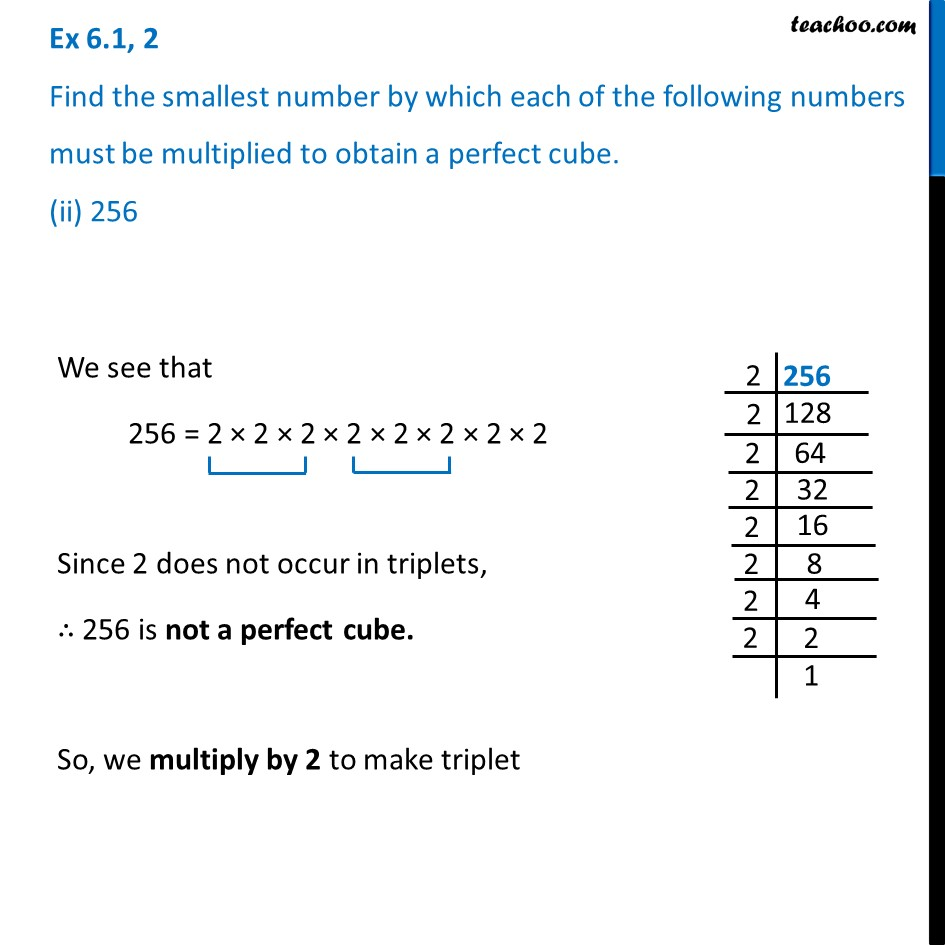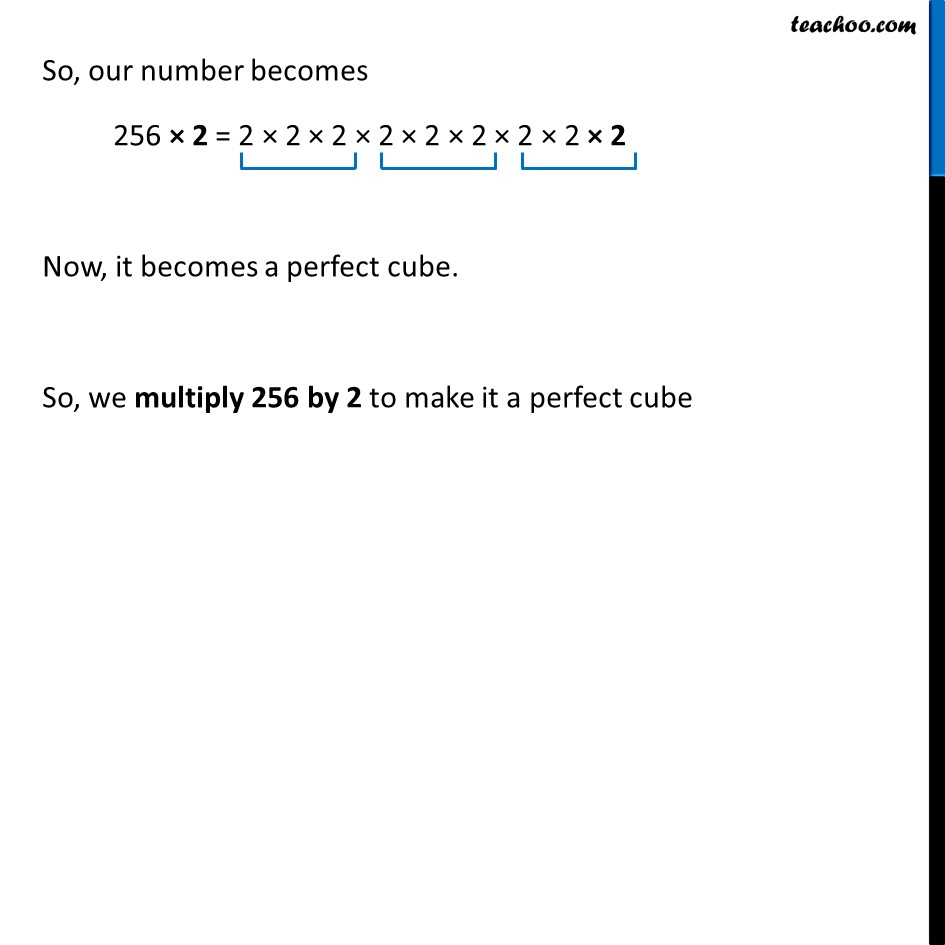Ex 6.1

Chapter 6 Class 8 Cubes and Cube Roots
Serial order wiseLearn in your speed, with individual attention - Teachoo Maths 1-on-1 Class

### Transcript

Ex 6.1, 2 Find the smallest number by which each of the following numbers must be multiplied to obtain a perfect cube. (ii) 256 We see that 256 = 2 × 2 × 2 × 2 × 2 × 2 × 2 × 2 Since 2 does not occur in triplets, ∴ 256 is not a perfect cube. So, we multiply by 2 to make triplet So, our number becomes 256 × 2 = 2 × 2 × 2 × 2 × 2 × 2 × 2 × 2 × 2 Now, it becomes a perfect cube. So, we multiply 256 by 2 to make it a perfect cube